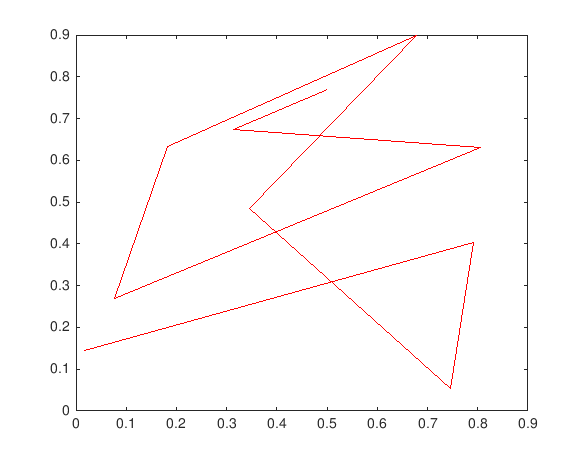Cody

# Problem 1744. Make a Plot with Functions

Solution 1590555

Submitted on 24 Jul 2018 by fbaillon
This solution is locked. To view this solution, you need to provide a solution of the same size or smaller.

### Test Suite

Test Status Code Input and Output
1   Pass
x = rand(10,1); y = rand(1,10); colors = ['g', 'r', 'b']; colorNum = randi(length(colors)); color = colors(colorNum); colorCodes = {[0 1 0], [1 0 0], [0 0 1]}; color_correct = colorCodes{colorNum}; h = makeAPlot(x,y,color); poltHandle = get(h); plotColor = poltHandle(1).Color; plotX = poltHandle(1).XData; plotY = poltHandle(1).YData; assert(isequal(plotColor,color_correct)) assert(isequal(plotX',x)) assert(isequal(plotY,y))

``

### Community Treasure Hunt

Find the treasures in MATLAB Central and discover how the community can help you!

Start Hunting!